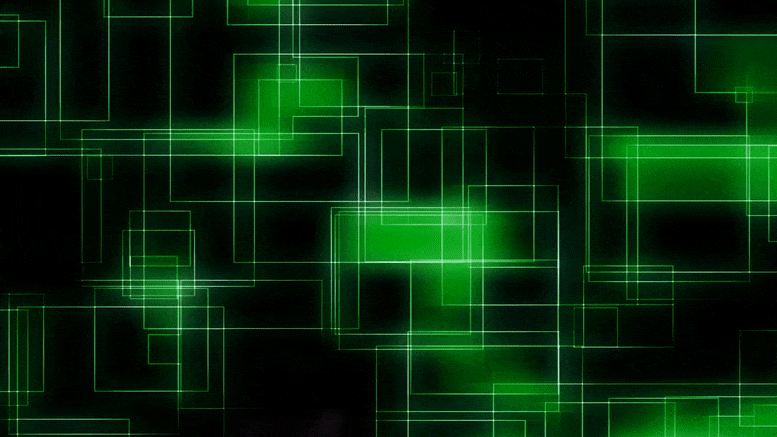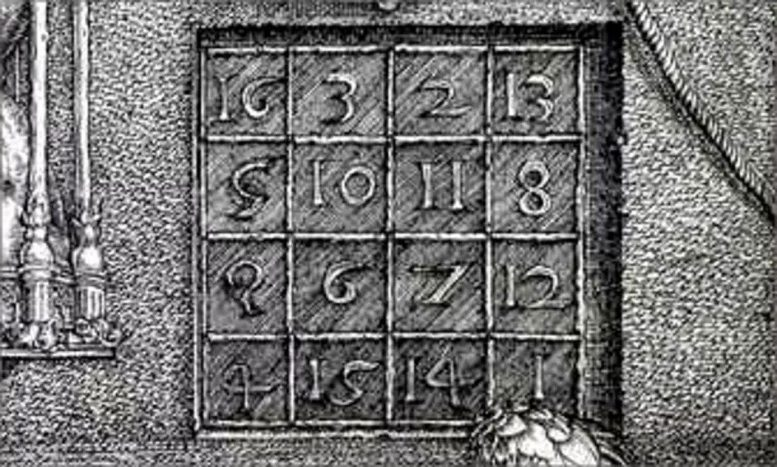# Quantum Magic Squares Cannot Be As Easily Characterized As Their “Classical” CousinsThe magic of mathematics is particularly reflected in magic squares. Recently, quantum physicist Gemma De las Cuevas and mathematicians Tim Netzer and Tom Drescher introduced the notion of the quantum magic square, and for the first time studied in detail the properties of this quantum version of magic squares.

Magic squares belong to the imagination of humanity for a long time. The oldest known magic square comes from China and is over 2000 years old. One of the most famous magic squares can be found in Albrecht Dürer’s copper engraving Melencolia I. Another one is on the facade of the Sagrada Família in Barcelona. A magic square is a square of numbers such that every column and every row sums to the same number. For example, in the magic square of the Sagrada Família every row and column sums to 33.Image: Detail from Melencolia I by Albrecht Dürer. Credit: National Library of Spain CC BY-NC-SA 4.0

If the magic square can contain real numbers, and every row and column sums to 1, then it is called a doubly stochastic matrix. One particular example would be a matrix that has 0’s everywhere except for one 1 in every column and every row. This is called a permutation matrix. A famous theorem says that every doubly stochastic matrix can be obtained as a convex combination of permutation matrices. In words, this means that permutation matrices “contain all the secrets” of doubly stochastic matrices—more precisely, that the latter can be fully characterized in terms of the former.

In a new paper in the Journal of Mathematical Physics, Tim Netzer and Tom Drescher from the Department of Mathematics and Gemma De las Cuevas from the Department of Theoretical Physics have introduced the notion of the quantum magic square, which is a magic square but instead of numbers, one puts in matrices. This is a non-commutative, and thus quantum, generalization of a magic square. The authors show that quantum magic squares cannot be as easily characterized as their “classical” cousins. More precisely, quantum magic squares are not convex combinations of quantum permutation matrices. “They are richer and more complicated to understand”, explains Tom Drescher. “This is the general theme when generalizations to the non-commutative case are studied.”

“The work is at the intersection of algebraic geometry and quantum information and showcases the benefits of interdisciplinary collaboration”, say Gemma De las Cuevas and Tim Netzer.

Reference: “Quantum magic squares: Dilations and their limitations featured” by Gemma De las Cuevas, Tom Drescher and Tim Netzer, 16 November 2020, Journal of Mathematical Physics.
DOI: 10.1063/5.0022344

#### 1 Commenton "Quantum Magic Squares Cannot Be As Easily Characterized As Their “Classical” Cousins"

1.xABBAAA | June 24, 2021 at 11:26 pm | Reply

… back to magic squares?